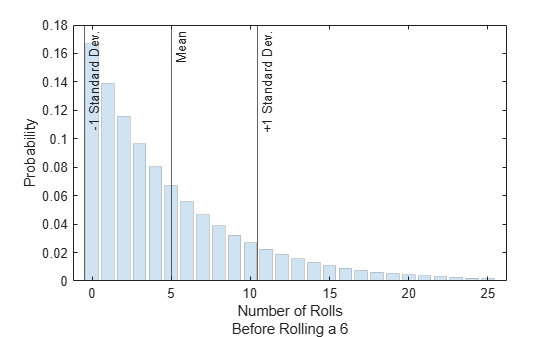# geostat

Geometric mean and variance

## Syntax

``[m,v] = geostat(p)``

## Description

example

````[m,v] = geostat(p)` returns the mean `m` and variance `v` of a geometric distribution with the corresponding probability parameter in `p`. For more information, see Geometric Distribution Mean and Variance.```

## Examples

collapse all

Roll a fair die repeatedly until you successfully get a 6. The associated geometric distribution models the number of times you roll the die before the result is a 6. Determine the mean and variance of the distribution, and visualize the results.

Because the die is fair, the probability of successfully rolling a 6 in any given trial is `p` = 1/6. Compute the mean and variance of the geometric distribution.

```p = 1/6; [m,v] = geostat(p)```
```m = 5.0000 ```
```v = 30.0000 ```

Notice that the mean `m` is $\left(1-p\right)/p$ and the variance `v` is $\left(1-p\right)/{p}^{2}$.

`m2 = (1-p)/p`
```m2 = 5.0000 ```
`v2 = (1-p)/p^2`
```v2 = 30.0000 ```

Evaluate the probability density function (pdf), or probability mass function (pmf), at the points `x` = 0,1,2,...,25.

```rng("default") % For reproducibility x = 0:25; y = geopdf(x,p);```

Plot the pdf values. Indicate the mean, one standard deviation below the mean, and one standard deviation above the mean.

```bar(x,y,"FaceAlpha",0.2,"EdgeAlpha",0.2); xline([m-sqrt(v) m m+sqrt(v)],"-", ... ["-1 Standard Dev.","Mean","+1 Standard Dev."]) xlabel(["Number of Rolls","Before Rolling a 6"]) ylabel("Probability")```Create a probability vector that contains three different parameter values.

• The first parameter corresponds to a geometric distribution that models the number of times you toss a coin before the result is heads.

• The second parameter corresponds to a geometric distribution that models the number of times you roll a four-sided die before the result is a 4.

• The third parameter corresponds to a geometric distribution that models the number of times you roll a six-sided die before the result is a 6.

`p = [1/2 1/4 1/6];`

Compute the mean and variance of each geometric distribution.

`[m,v] = geostat(p)`
```m = 1×3 1.0000 3.0000 5.0000 ```
```v = 1×3 2.0000 12.0000 30.0000 ```

The returned values indicate that, for example, the mean of a geometric distribution with probability parameter `p` = 1/4 is 3, and the variance of the distribution is 12.

## Input Arguments

collapse all

Probability of success in a single trial, specified as a scalar or an array of scalars in the range [0,1]. To compute the means and variances of multiple distributions, specify the distribution parameters `p` using an array of scalar values.

Example: `0.5`

Example: `[1/2 1/3]`

Data Types: `single` | `double`

## Output Arguments

collapse all

Mean of the geometric distribution, returned as a numeric scalar or an array of numeric scalars. `m` is the same size as `p`, and each element in `m` is the mean of the geometric distribution specified by the corresponding element in `p`.

Variance of the geometric distribution, returned as a numeric scalar or an array of numeric scalars. `v` is the same size as `p`, and each element in `v` is the variance of the geometric distribution specified by the corresponding element in `p`.

The mean of the geometric distribution is $\text{mean}=\frac{1-p}{p}\text{\hspace{0.17em}},$ and the variance of the geometric distribution is $\mathrm{var}=\frac{1-p}{{p}^{2}}\text{\hspace{0.17em}},$ where p is the probability of success.